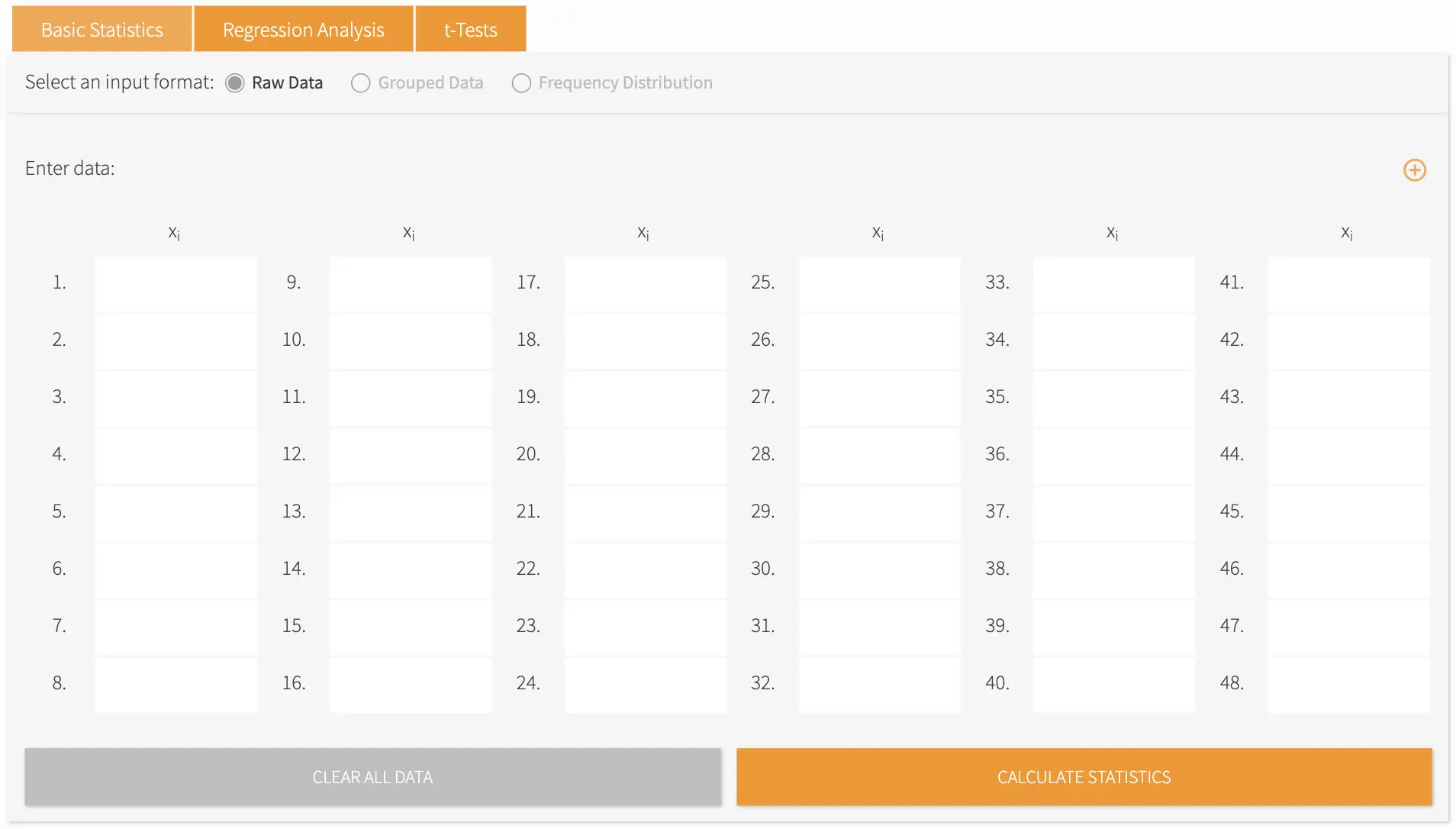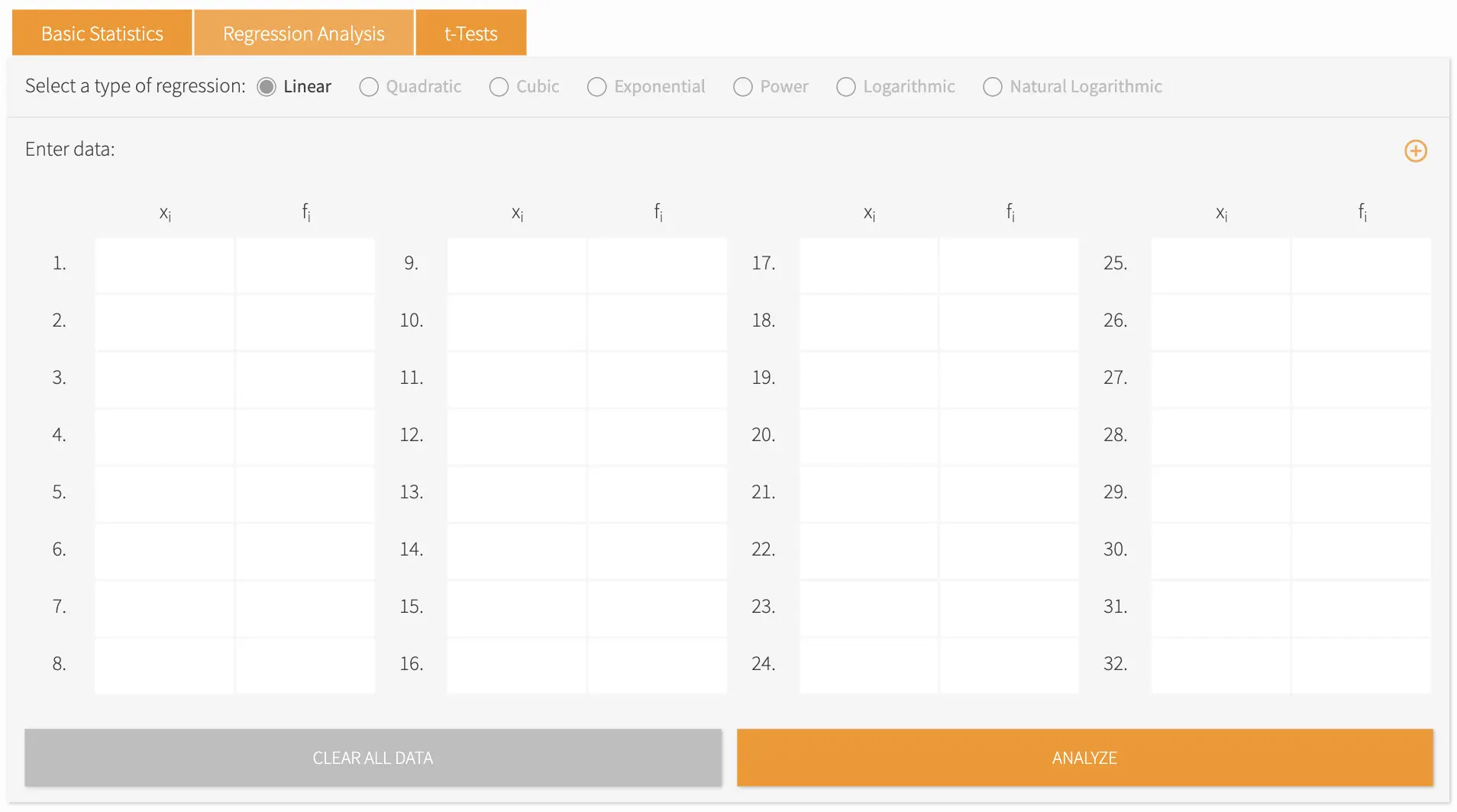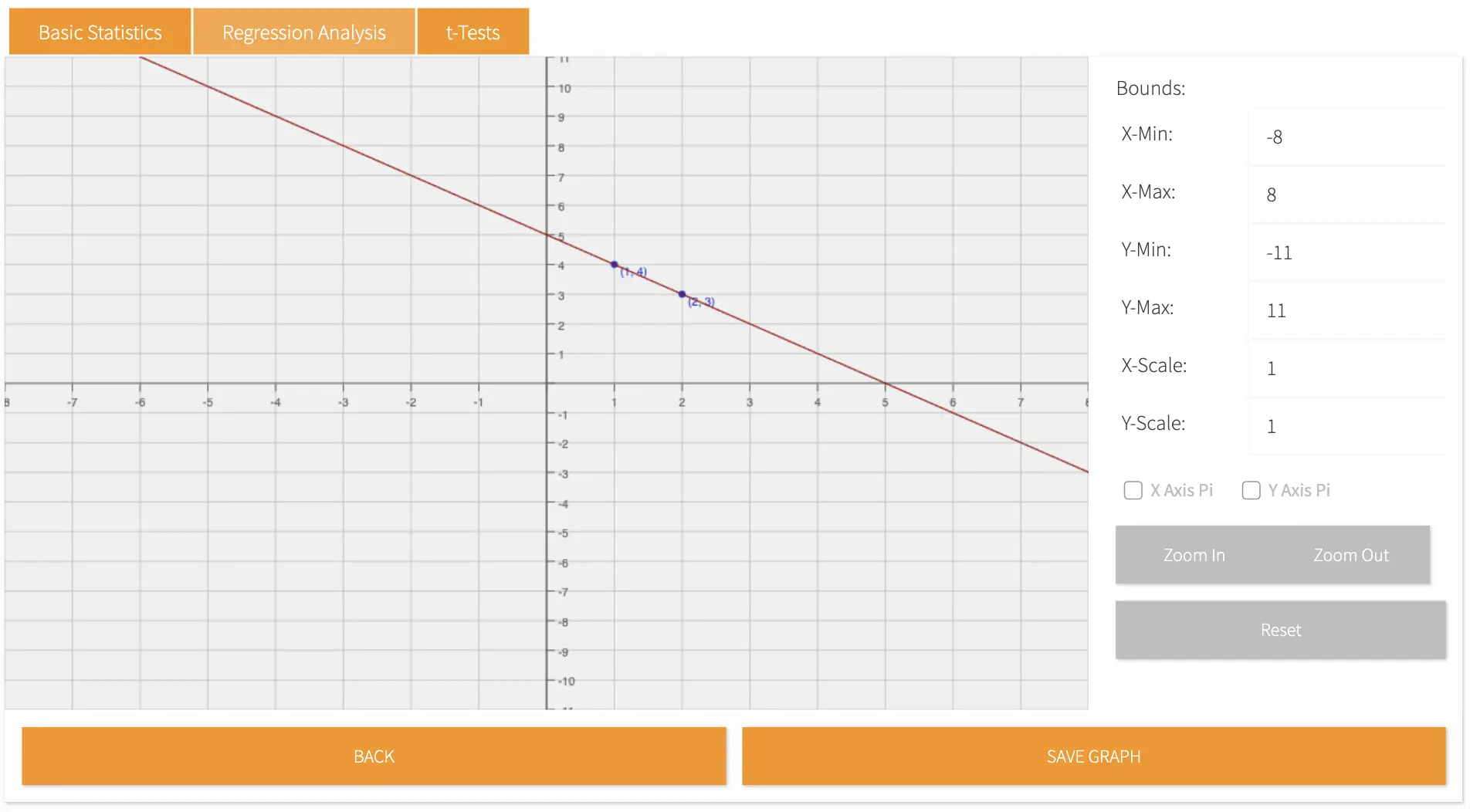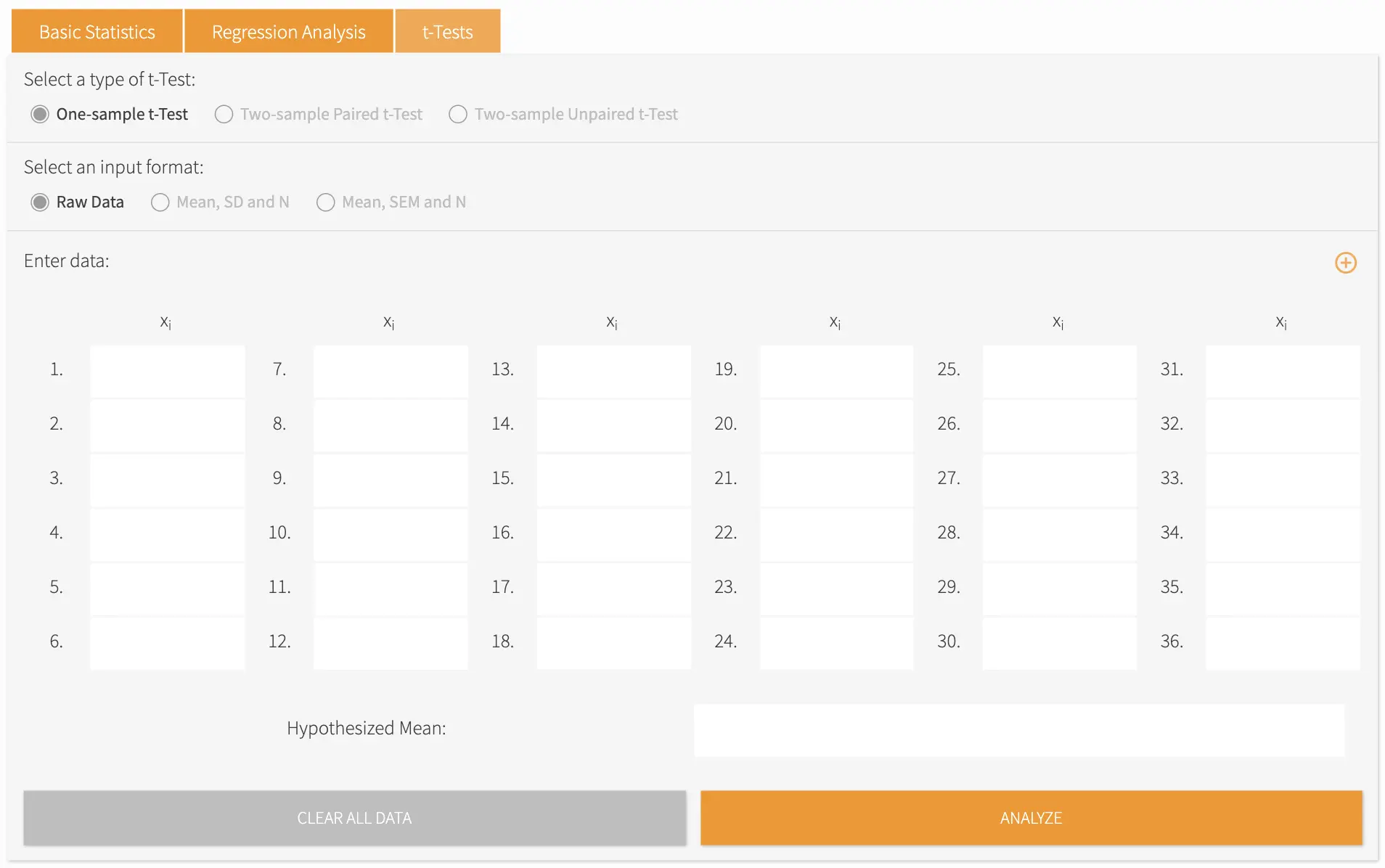# Statistics Calculator

• Our statistics calculator is the most sophisticated statistics calculator online.
• It can do all the basics like calculating quartiles, mean, median, mode, variance, standard deviation as well as the correlation coefficient.
• You can also do almost any kind of regression analysis (linear, quadratic, exponential, cubic , power, logarithmic and natural Logarithmic). The regressions and points can then be graphed.
• Can Compute student 1 or 2-Tailed T-Tests (paired and unpaired). You can enter raw data and then calculate T-tests.# Best Statistics Calculator Online

Method:
This operation is taking too long to respond.
-

Statistics is a subfield of mathematics that is involved with the gathering, examination, interpretation, and presentation of large quantities of numerical data. Large-scale numerical data collection and analysis, particularly with the aim of determining proportions in the whole from those in a representative sample, constitute the activity or science of statistics.

Statistics is frequently used to understand natural events. When applying statistics to a scientific, industrial, or social problem, it is typical to start with a statistical population or a statistical model that will be evaluated.

By using our comprehensive online statistics calculator, you can determine the mean, median, mode, standard deviation, variance, or correlation coefficient of any given dataset.Figure 1. Screenshot of the Basic Statistics Calculator

Practically any type of regression analysis is possible such as linear, quadratic, exponential, cubic, power, logarithmic and natural logarithmic. You can then graph the points and regressions using our online tool.Figure 2. Screenshot of the Regression Analysis CalculatorFigure 3. Screenshot of a sample plotted graph that can be saved using the Save button

Our online statistics calculator can also calculate paired and unpaired t-tests. All you have to do is select the type of test and enter the chosen data format in the fields.Figure 4. Screenshot of the t-Test Calculator